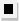Chapter 13.6, Problem 47E### Algebra and Trigonometry (MindTap ...

4th Edition
James Stewart + 2 others
ISBN: 9781305071742

#### Solutions

Chapter
Section### Algebra and Trigonometry (MindTap ...

4th Edition
James Stewart + 2 others
ISBN: 9781305071742
Textbook Problem

# 4 7 - 4 8Simplifying a Difference Quotient Simplify using the Binomial Theorem. ( x + h ) 3 − x 3 h

To determine

To find:

Simplify (x+h)3x3h using Binomial theorem

Explanation

Given:

The expression (x+h)3x3h

Approach:

Expand the binomial term using binomial expansion and simplify.

Calculation:

(x+h)3x3h=x3+3x2h+3x

### Still sussing out bartleby?

Check out a sample textbook solution.

See a sample solution

#### The Solution to Your Study Problems

Bartleby provides explanations to thousands of textbook problems written by our experts, many with advanced degrees!

Get Started